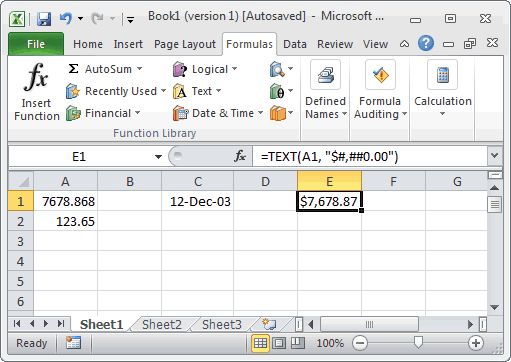# How to use the TEXT Function (WS)This Excel tutorial explains how to use the Excel TEXT function with syntax and examples.

## Description

The Microsoft Excel TEXT function returns a value converted to text with a specified format.

The TEXT function is a built-in function in Excel that is categorized as a String/Text Function. It can be used as a worksheet function (WS) in Excel. As a worksheet function, the TEXT function can be entered as part of a formula in a cell of a worksheet.

## Syntax

The syntax for the TEXT function in Microsoft Excel is:

`TEXT( value, format )`

### Parameters or Arguments

value
The value to convert to text.
format
The format used to display the result.

## Applies To

• Excel 2016, Excel 2013, Excel 2011 for Mac, Excel 2010, Excel 2007, Excel 2003, Excel XP, Excel 2000

## Type of Function

• Worksheet function (WS)

## Example (as Worksheet Function)

Let’s look at some Excel TEXT function examples and explore how to use the TEXT function as a worksheet function in Microsoft Excel:Based on the Excel spreadsheet above, the following TEXT examples would return:

```=TEXT(A1, "\$#,##0.00")
Result: "\$7,678.87"

=TEXT(A1, "0")
Result: "7679"

=TEXT(A2, "0.0")
Result: "123.7"

=TEXT(1277, "#,##0")
Result: "1,277"

=TEXT(C1, "yyyy/mm/dd")
Result: "2003/12/12"

=TEXT(C1, "mmm dd, yyyy")
Result: "Dec 12, 2003"```